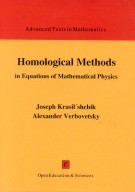## Homological methods in equations of mathematical physics

#### byI. S. Krasil'shchik  and  A. M. Verbovetsky

Open Education, Opava, 1998, 150 pp.
Contents

Introduction
1 Differential calculus over commutative algebras
1.1 Linear differential operators
1.2 Multiderivations and the Diff-Spencer complex
1.3 Jets
1.4 Compatibility complex
1.5 Differential forms and the de Rham complex
1.6 Left and right differential modules
1.7 The Spencer cohomology
1.8 Geometrical modules
2 Algebraic model for Lagrangian formalism
2.2 Berezinian and integration
2.3 Green's formula
2.4 The Euler operator
2.5 Conservation laws
3 Jets and nonlinear differential equations. Symmetries
3.1 Finite jets
3.2 Nonlinear differential operators
3.3 Infinite jets
3.4 Nonlinear equations and their solutions
3.5 Cartan distribution on Jk(p)
3.6 Classical symmetries
3.7 Prolongations of differential equations
3.8 Basic structures on infinite prolongations
3.9 Higher symmetries
4 Coverings and nonlocal symmetries
4.1 Coverings
4.3 Reconstruction theorems
5 Fröliher-Nijenhuis brackets and recursion operators
5.1 Calculus in form-valued derivations
5.2 Algebras with flat connections and cohomology
5.3 Applications to differential equations: recursion operators
5.4 Passing to nonlocalities
6 Horizontal cohomology
6.1 C-modules on differential equations
6.2 The horizontal de Rham complex
6.3 Horizontal compatibility complex
6.4 Applications to computing the C-cohomology groups
6.5 Example: Evolution equations